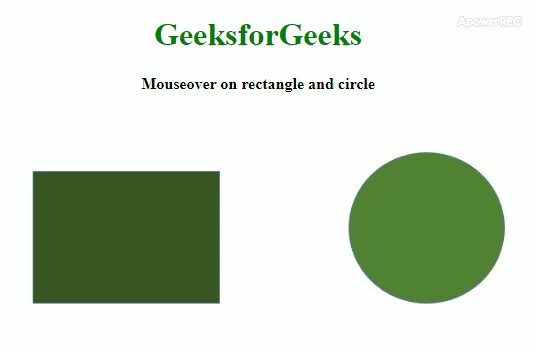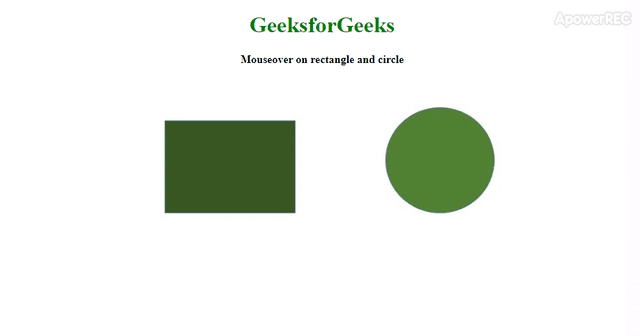Related Articles
ES6 | Image Map
• Last Updated : 20 Mar, 2020

Image map in JavaScript is used to navigate various links to other web pages or some sections of the same web page from any image on that web page. Which is let an image on a web page, this image divided into several sections, each section is corresponding to some links, after clicking on those section of that image, the links are opened. This sections of image is refer as image map. This sections have different shapes such as rectangle, circle etc. The image is inserted into the web page using <img> tag and under this tag there is a attribute called usemap which defines the map description into that image. In simple words, the usemap attribute calls the map description which is applied to the image. This map is defined in such a way that the image is divided into some sections. These sections are defined by specifying coordinates of pixels, which defines the boundaries of each clickable sections. There is an another tag <area> which is defined under the map. Under this <area> tag the coordinates and the shapes of each section are defined. This sections are referred as hotspot.

Syntax:

```<img src="GFG.jpg" usemap=#mapName>
<map name="mapName">
<area shape=shapeName coords="x, y" />
<area shape=shapeName coords="x, y, r" />
</map>```

Below example illustrate the image map in ES6:

• Example:
 ```<``html``>``<``head``>``    ``<``script` `type``=``"text/javascript"``>``        ``function fn(name) {``            ``document.getElementById("typ").innerHTML = name;``        ``}``    `````` ` `<``body``>``    ``<``center``>``        ``<``h1` `style``=``"color:green;"``>GeeksforGeeks``        ``<``b``>Mouseover on rectangle and circle``        ``<``br``>``    ``<``span` `id``=``"typ"` `style``=``"color:#008000;"``>``    ``<``br``>``    ``<``img` `src``=``"https://media.geeksforgeeks.org/wp-content/uploads/20200309123213/sample16.png"``         ``width``=``"600"` `height``=``"auto"` `usemap=#gfg>``   ` `    ````    ``<``map` `name``=``"gfg"``>``         ` `        ````        ````        ``<``area` `shape``=``"rect"` `coords``=``"75, 60, 250, 200"``              ``onmouseover``=``"fn('RECTANGLE')"` `              ``onmouseout``=``"fn('')"` `/>``        ``<``area` `shape``=``"circle"` `              ``coords``=``"500, 80, 110"` `              ``onmouseover``=``"fn('CIRCLE')"` `              ``onmouseout``=``"fn('')"``>``    `````` ` ``

Output: In this code coordinate 75, 60, 250, 200 refers to the rectangle and coordinate 500, 80, 110 refers to the circle. In the image the sections corresponding to the coordinates are created, into which the events are happen.• Example 2: In this example, we will open a link using image map.
 ```<``html``>``<``head``>``    ``<``script` `type``=``"text/javascript"``>``        ``function fn(name) {``            ``document.getElementById("typ").innerHTML = name;``        ``}``    `````` ` `<``body``>``    ``<``center``>``        ``<``h1` `style``=``"color:green;"``>GeeksforGeeks``        ``<``b``>Mouseover on rectangle and circle``        ``<``br``>``    ``<``span` `id``=``"typ"` `style``=``"color:#008000;"``>``    ``<``br``>``    ``<``img` `src``=``"https://media.geeksforgeeks.org/wp-content/uploads/20200309123213/sample16.png"``         ``width``=``"600"` `height``=``"auto"` `usemap=#gfg>``   ` `    ````    ``<``map` `name``=``"gfg"``>``         ` `        ````        ````        ``<``area` `shape``=``"rect"` `coords``=``"75, 60, 250, 200"``              ``href``=``"https://ide.geeksforgeeks.org/tryit.php"``              ``onmouseover``=``"fn('RECTANGLE')"` `              ``onmouseout``=``"fn('')"` `/>``        ``<``area` `shape``=``"circle"` `              ``coords``=``"500, 80, 110"` `              ``onmouseover``=``"fn('CIRCLE')"` `              ``onmouseout``=``"fn('')"``>``    `````` ` `           `
• Output:My Personal Notes arrow_drop_up